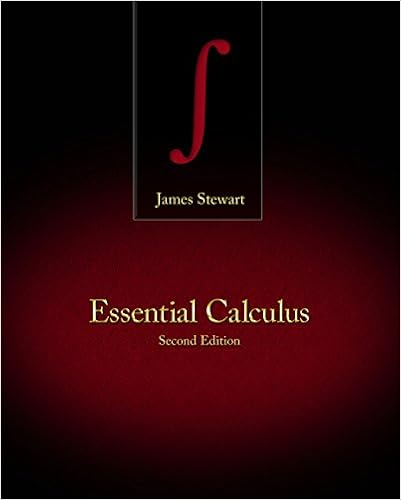# Lab 2 - Lab 2 Mapping of Electric Fields Written by Stefan...

• Lab Report
• 6

This preview shows page 1 - 3 out of 6 pages.

##### We have textbook solutions for you!
The document you are viewing contains questions related to this textbook.The document you are viewing contains questions related to this textbook.
Chapter 10 / Exercise 48
Essential Calculus
StewartExpert Verified
Lab 2Mapping of Electric FieldsWritten by: Stefan JenkinsPerformed: 1/19/2012Conducted with: Richard MelsonGreg Newman
##### We have textbook solutions for you!
The document you are viewing contains questions related to this textbook.The document you are viewing contains questions related to this textbook.
Chapter 10 / Exercise 48
Essential Calculus
StewartExpert Verified
Introduction & Purpose of ExperimentAs stated in the lab manual, an electric field is a region in which forces of electrical origin are exerted on any electric charges that may be present. The ratio of force(F) to charge (q) is represented by field strength (E). Force is a vector quantity and chargeis a scalar quantity; therefore the resulting field strength is also a vector quantity. This relationship is described in equation 1. (1)For a charge (q) to travel to a new location in the field, electrical work (W)must be expended. The ratio of ‘the work required to move the charge’ and ‘the magnitude of the charge’ is equal to the potential difference, or voltage (V) between the two locations. (2)Within the field, two points may have a potential difference of zero. Summing a series of points that have a potential difference of zero results in an equipotential line. Lines of force in an electric field run perpendicular to equipotential lines. This experiment maps equipotential lines in an attempt to map the lines of force.
•••